# ggrastr

### Convert any ggplot2 layer into a rasterized output

Using the function `rasterize()`, users can rasterize any ggplot2 layer:

``````library(ggplot2)
library(ggrastr)

plot <- ggplot(diamonds, aes(carat, price, colour = cut))

plot + rasterise(geom_point(), dpi = 72) + theme(aspect.ratio = 1)``````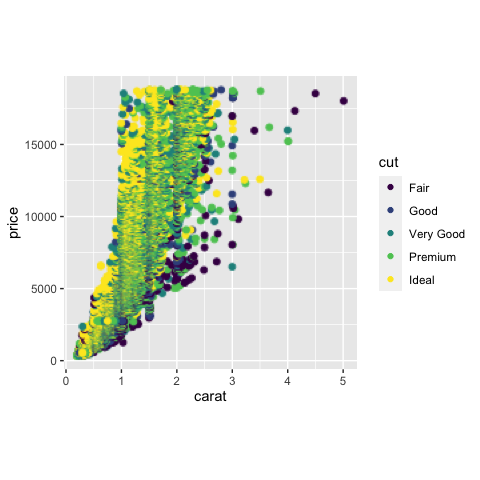Note that with ggrastr changes in version 0.2.0, when the aspect ratio is distorted, the objects are rendered without distortion, i.e. the points in this example are still circles:

``````# Points remain round across different aspect ratios
plot + rasterise(geom_point(), dpi = 72) + theme(aspect.ratio = 0.2)``````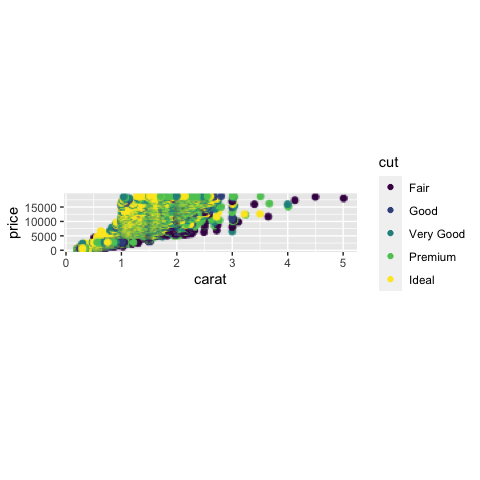By default, plots are rendered with Cairo. However, users now have the option to render plots with the ragg device. The motivation for using `ragg` is that `ragg` can be faster and has better anti-aliasing. That being said, the default ragg device also has some alpha blending quirks. Because of these quirks, users are recommended to use the `ragg_png` option to work around the alpha blending.

The differences in devices are best seen at lower resolution:

``````# The default 'cairo' at dpi=5
plot + rasterise(geom_point(), dpi = 5, dev = "cairo")``````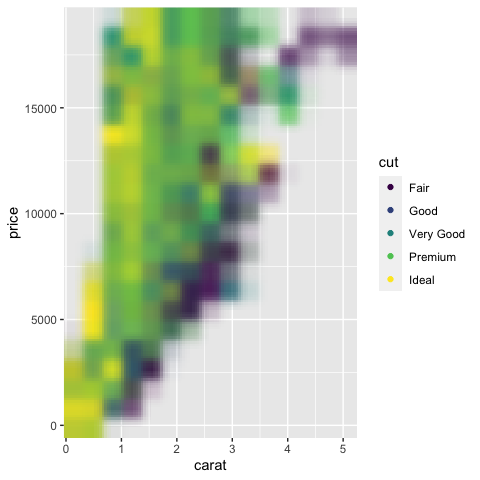``````# Using 'ragg' gives better anti-aliasing but has unexpected alpha blending
plot + rasterise(geom_point(), dpi = 5, dev = "ragg")``````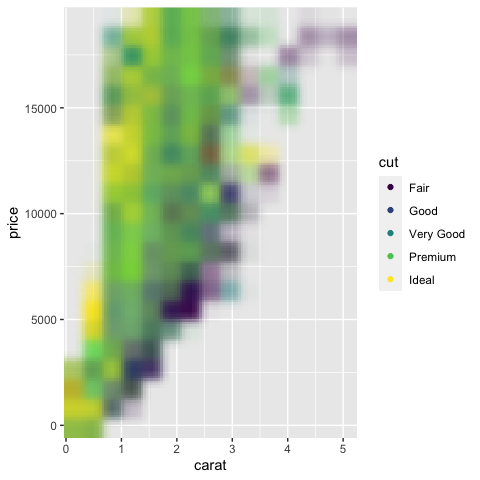``````# Using 'ragg_png' solves the alpha blend, but requires writing a temporary file to disk
plot + rasterise(geom_point(), dpi = 5, dev = "ragg_png")``````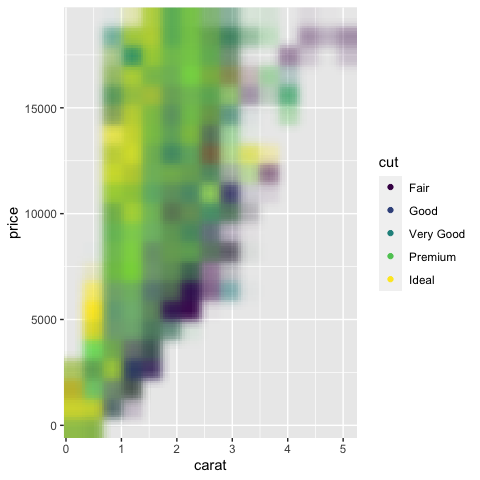### Raserized plots with facet_wrap()

Facets are rendered correctly without users having to adjust the width/height settings.

``````# Facets will not warp/distort points
set.seed(123)
plot + rasterise(geom_point(), dpi = 300) + facet_wrap(~ sample(1:3, nrow(diamonds), 2))``````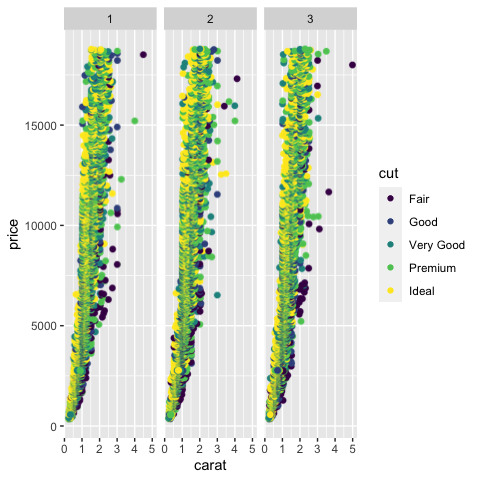### Scaling size of raster objects

Users are also able to change the size of the raster objects with the parameter `scale`. The default behavior is not to modify the size with `scale=1`:

``````# unchanged scaling, scale=1
plot <- ggplot(diamonds, aes(carat, price, colour = cut))
plot + rasterise(geom_point(), dpi = 300, scale = 1)``````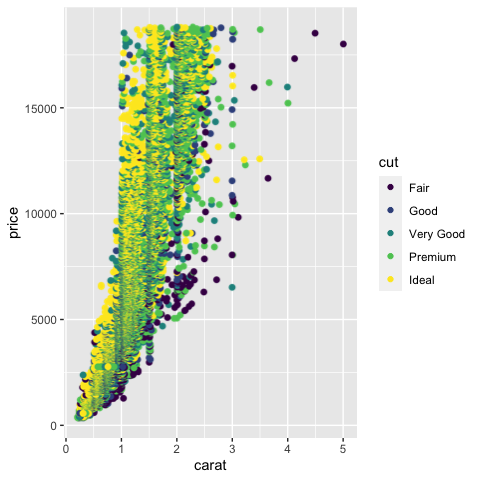Setting `scale` to values greater than 1 will increase the size of the rasterized objects. In this case, `scale=2` will double the size of the points in comparison to the original plot:

``````# larger objects, scale > 1
plot <- ggplot(diamonds, aes(carat, price, colour = cut))
plot + rasterise(geom_point(), dpi = 300, scale = 2)``````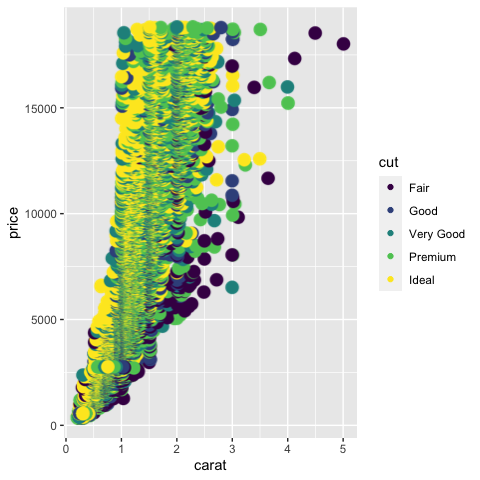Similarly, values less than 1 will result in smaller objects. Here we see `scale=0.5` results in points half the size of the points in the original plot above:

``````# smaller objects, scale < 1
plot <- ggplot(diamonds, aes(carat, price, colour = cut))
plot + rasterise(geom_point(), dpi = 300, scale = 0.5)``````